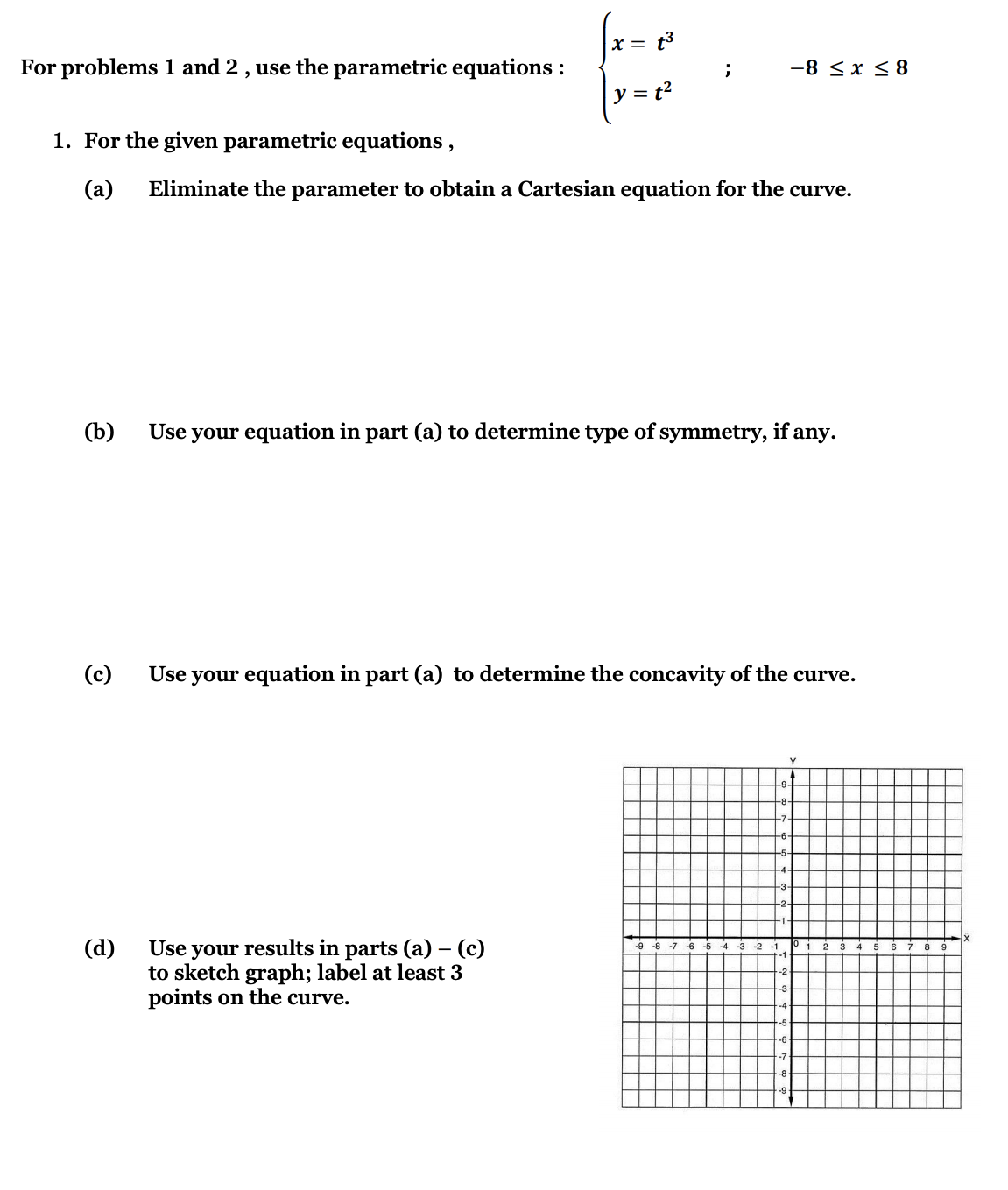YY

Yaboiimarco Y.

Calculus 2 / BC

5 days, 10 hours ago

All problems step by stepAll problems step by step

### Discussion

You must be signed in to discuss.

### Video Transcript

okay if we sketched thes two are metrics. We're gonna make a chart with time and then our X value and are y value. So let's start with time zero and you put zero in for tea and the ex equation. You'll get one, and you put it in the Why equation and you will get 1/4 about one in for tea. Three for X. Yeah, let's see one in for tea. It's 1.5 3 have squared. Is 9/4. That's putting it, too. Uh, that's a B A four plus one. We have five. We have 2.5, which is five have. So that's 25 4th We'll go three six. We got seven here. 3.5 That's 7/2 sets. 49 4th Let's see if we're going to go any further. Yeah, it's probably good right there. Stop with that and you can keep outing. More values for tea if you'd like to get more points, but we'll go ahead. Graph what we have. We're gonna graphing X's and y's together. So we have one and forth three and no fourth, which is 2.25 Just a little about the to five and 25 forces. This six and 1/4 just about six and seven and 49 Force, which is 12.45 Which puts me a little bit off the grid there. 11. So be up there a little bit, and then we can crap those like So now that would keep going upwards. And you can even mark sometimes in here if you would want, like, t equals zero right there. Teeples to right there. Okay, then you want to have a rectangular equation. So let's take our two per metrics. You want to solve one of these for tea, which the X equation will probably easier to solve for t divide both sides by two. That would give you 1/2 X minus 1/2 equals. T says INSAT equals T. We're going to replace or sub that in for tea and the other equation, so we're gonna have 1/2 x minus 1/2 plus 1/2 squared. We can simplify that. The two halfs cancel. So we have 1/2 x squared and we square the half That's 1/4##### Top Calculus 2 / BC Educators##### Catherine R.

Missouri State University##### Michael J.

Idaho State University